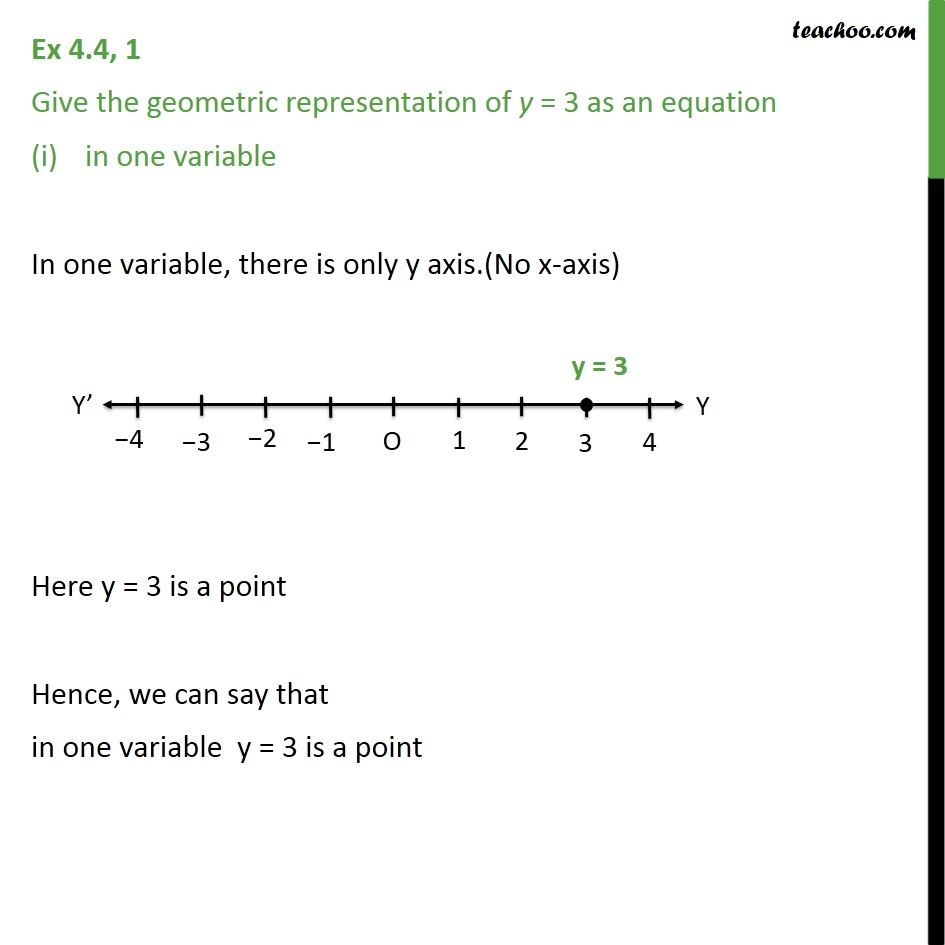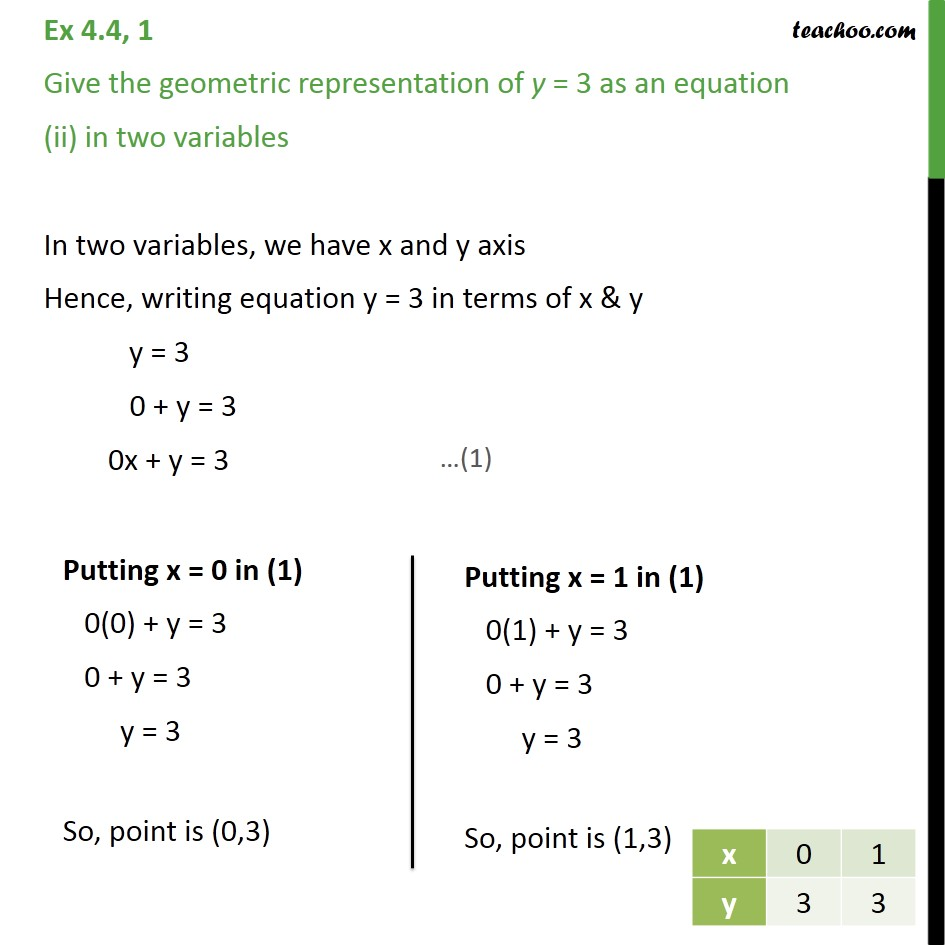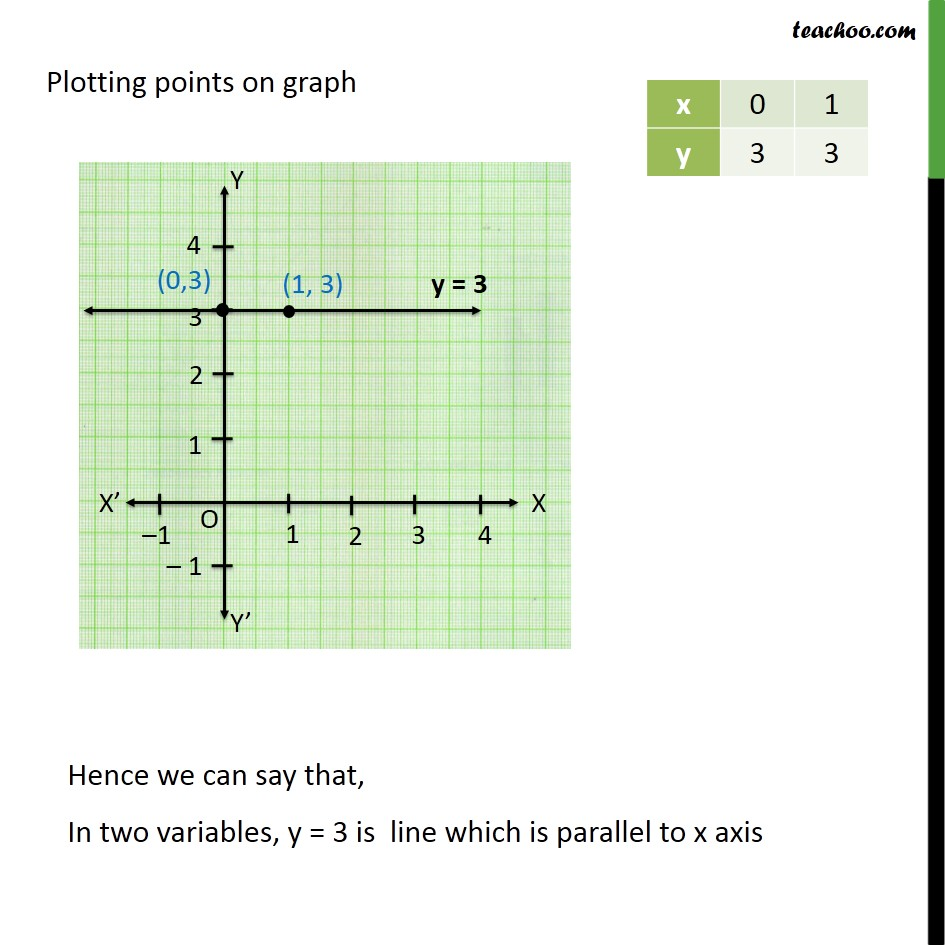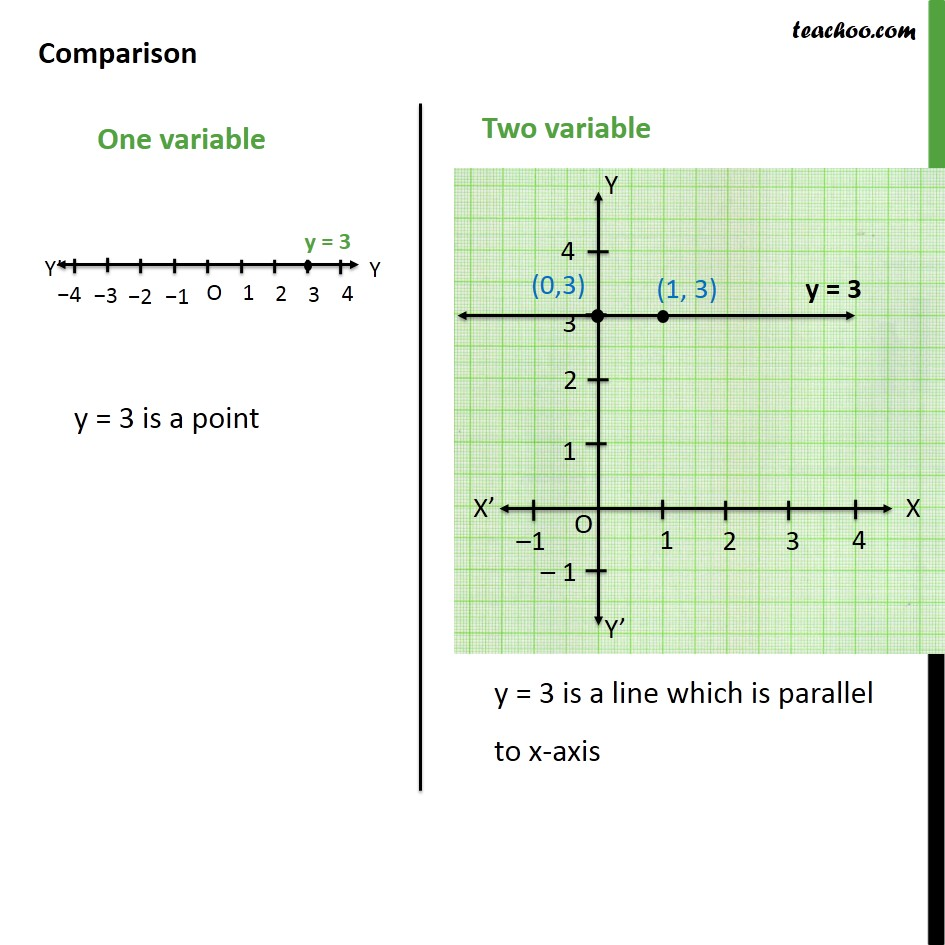Subscribe to our Youtube Channel - https://you.tube/teachoo

1. Class 9
2. Important Questions for Exam - Class 9
3. Chapter 4 Class 9 Linear Equations in Two Variables

Transcript

Ex 4.4, 1 Give the geometric representation of y = 3 as an equation in one variable In one variable, there is only y axis.(No x-axis) Here y = 3 is a point Hence, we can say that in one variable y = 3 is a point Ex 4.4, 1 Give the geometric representation of y = 3 as an equation (ii) in two variables In two variables, we have x and y axis Hence, writing equation y = 3 in terms of x & y y = 3 0 + y = 3 0x + y = 3 Plotting points on graph Comparison

Chapter 4 Class 9 Linear Equations in Two Variables

Class 9
Important Questions for Exam - Class 9